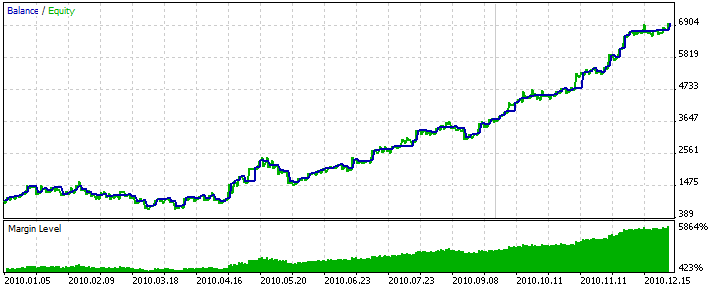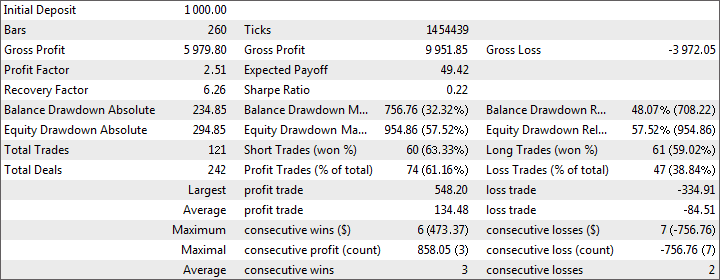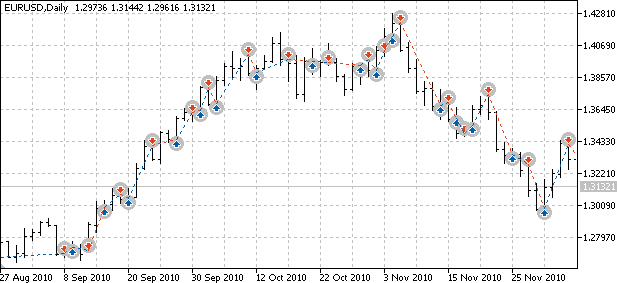MT5连接 NeuroSolutions 神经网络 - 小众知识

# MT5连接 NeuroSolutions 神经网络

2013年01月27日 14:18:05
标签： 神经网络

### 准备训练数据

```#property script_show_inputs
//+------------------------------------------------------------------+
input string    Export_FileName = "NeuroSolutions\\data.csv"; // File for exporting (in the folder "MQL5\Files")
input int       Export_Bars     = 260; // Number of lines to be exported
//+------------------------------------------------------------------+
void OnStart()
{

// Create the file
int file = FileOpen(Export_FileName, FILE_WRITE|FILE_CSV|FILE_ANSI, ',');

if (file != INVALID_HANDLE)
{
// Write the heading of data

string row="";
for (int i=0; i<=5; i++)
{
if (StringLen(row)) row += ",";
row += "Open"+i+",High"+i+",Low"+i+",Close"+i;
}
FileWrite(file, row);

// Copy all required information from the history

MqlRates rates[], rate;
int count = Export_Bars + 5;
if (CopyRates(Symbol(), Period(), 1, count, rates) < count)
{
Print("Error! Not enough history for exporting of data.");
return;
}
ArraySetAsSeries(rates, true);

// Write data

for (int bar=0; bar<Export_Bars; bar++)
{
row="";
double zlevel=0;
for (int i=0; i<=5; i++)
{
if (StringLen(row)) row += ",";
rate = rates[bar+i];
if (i==0) zlevel = rate.open; // level for counting of prices
row += NormalizeDouble(rate.open -zlevel, Digits()) + ","
+ NormalizeDouble(rate.high -zlevel, Digits()) + ","
+ NormalizeDouble(rate.low  -zlevel, Digits()) + ","
+ NormalizeDouble(rate.close-zlevel, Digits());
}
FileWrite(file, row);
}

FileClose(file);
Print("Export of data finished successfully.");
}
else Print("Error! Failed to create the file for data export. ", GetLastError());
}
//+------------------------------------------------------------------+```

```Open0,High0,Low0,Close0,Open1,High1,Low1,Close1,Open2,High2,Low2,Close2,Open3,High3,Low3,Close3,Open4,High4,Low4,Close4,Open5,High5,Low5,Close5
0,0.00463,-0.0041,0.00274,-0.00518,0.00182,-0.00721,-6e-005,0.00561,0.00749,-0.00413,-0.00402,0.02038,0.02242,0.00377,0.00565,0.03642,0.0379,0.01798,0.02028,0.0405,0.04873,0.03462,0.03647
0,0.007,-0.00203,0.00512,0.01079,0.01267,0.00105,0.00116,0.02556,0.0276,0.00895,0.01083,0.0416,0.04308,0.02316,0.02546,0.04568,0.05391,0.0398,0.04165,0.04504,0.05006,0.03562,0.0456
0,0.00188,-0.00974,-0.00963,0.01477,0.01681,-0.00184,4e-005,0.03081,0.03229,0.01237,0.01467,0.03489,0.04312,0.02901,0.03086,0.03425,0.03927,0.02483,0.03481,0.02883,0.04205,0.02845,0.03809
```

### 创建神经网络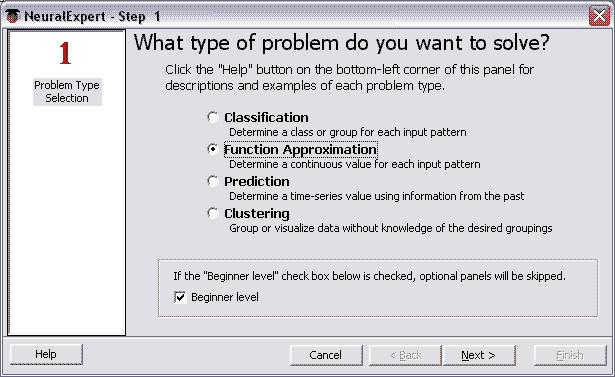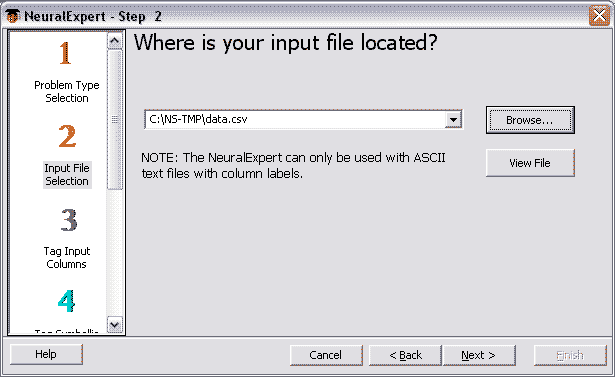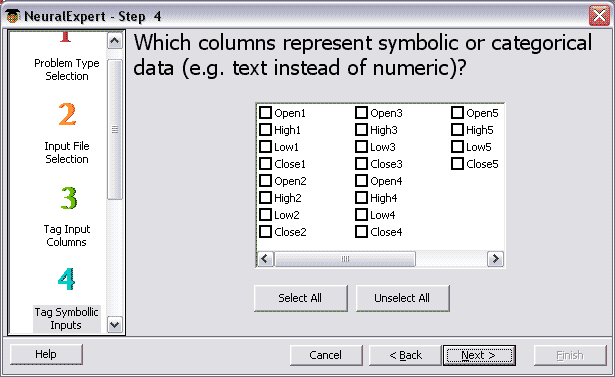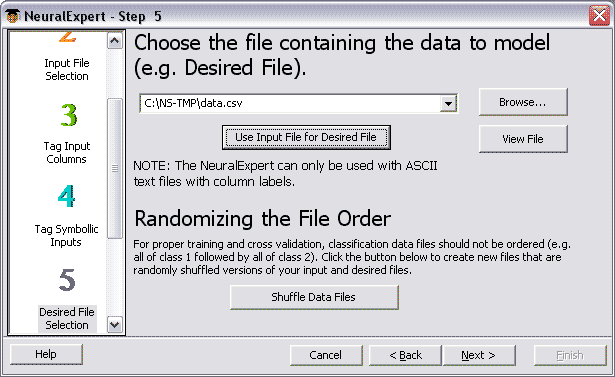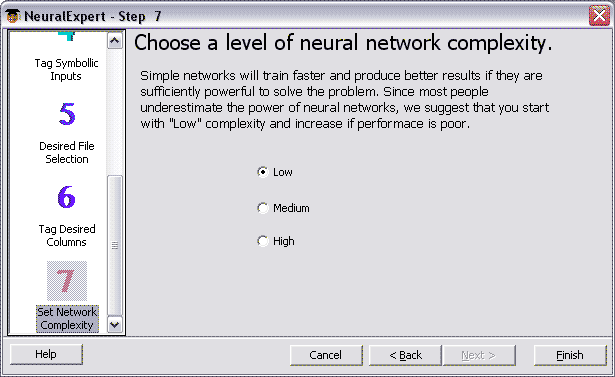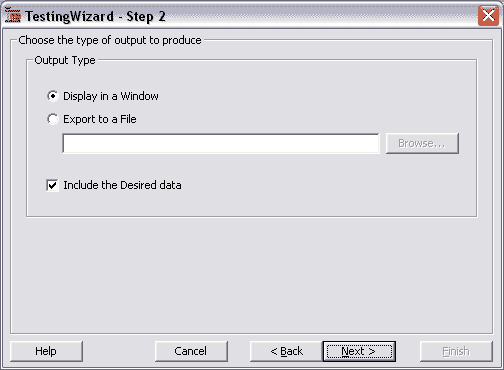### 将神经网络以 DLL 导出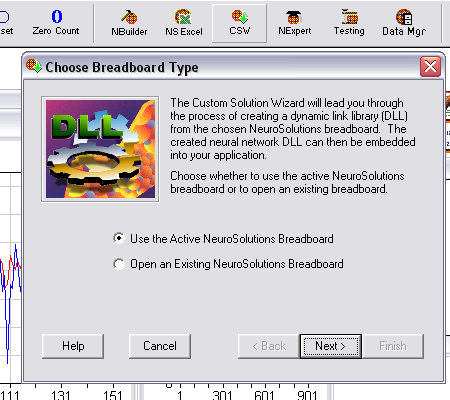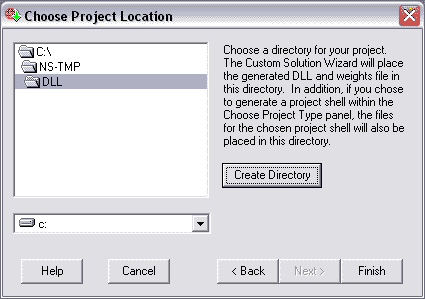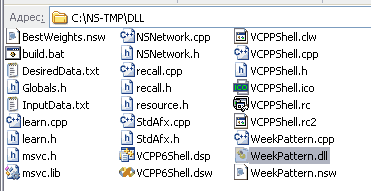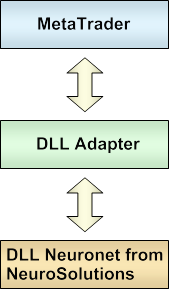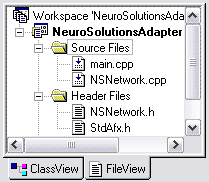```#include "stdafx.h"
#include "NSNetwork.h"

extern "C" __declspec(dllexport) int __stdcall CalcNeuralNet(
LPCWSTR dllPath_u, LPCWSTR weightsPath_u,
double* inputs, double* outputs)
{
// Transform the lines from Unicode to normal ones
CString dllPath     (dllPath_u);
CString weightsPath (weightsPath_u);

// Create neuronet
NSRecallNetwork nn(dllPath);

if (nn.LoadWeights(weightsPath) != 0) return (2);

// Pass input data and calculate the output
if (nn.GetResponse(1, inputs, outputs) != 0) return (3);

return 0;
}```

### 在“EA 交易”中使用神经网络

WeekPattern.dll 我们在 NeuroSolutions 中创建的 DLL 神经网络 MQL5\Files\NeuroSolutions\
WeekPattern.nsw 神经网络的平衡设置 MQL5\Files\NeuroSolutions\
NeuroSolutionsAdapter.dll 可用于任何 DLL 神经网络的通用 DLL 适配程序 MQL5\Libraries\

```input double    Lots = 0.1;
//+------------------------------------------------------------------+
// Connect the DLL adapter, using which we are going to use the DLL neuronet created in NeuroSolutions
int CalcNeuralNet(string dllPath, string weightsPath, double& inputs[], double& outputs[]);
#import
//+------------------------------------------------------------------+
class CNeuroSolutionsNeuralNet
{
private:
string dllPath;     // Path to a DLL neuronet created in NeuroSolutions
string weightsPath; // Path to a file of the neuronet balances
public:
double in; // Neuronet inputs - OHLC of 5 bars
double out; // Neuronet outputs - Close of a current bar

CNeuroSolutionsNeuralNet();
bool Calc();
};
//+------------------------------------------------------------------+
void CNeuroSolutionsNeuralNet::CNeuroSolutionsNeuralNet()
{
string terminal = TerminalInfoString(TERMINAL_PATH);
dllPath     = terminal + "\\MQL5\\Files\\NeuroSolutions\\WeekPattern.dll";
weightsPath = terminal + "\\MQL5\\Files\\NeuroSolutions\\WeekPattern.nsw";
}
//+------------------------------------------------------------------+
bool CNeuroSolutionsNeuralNet::Calc()
{
// Get current quotes for the neuronet
MqlRates rates[], rate;
CopyRates(Symbol(), Period(), 0, 6, rates);
ArraySetAsSeries(rates, true);

// Fill the array of input data of the neuronet
double zlevel=0;
for (int bar=0; bar<=5; bar++)
{
rate = rates[bar];
// 0 bar is not taken for input
if (bar==0) zlevel=rate.open; // level of price calculation
// 1-5 bars are inputed
else
{
int i=(bar-1)*4; // input number
in[i  ] = rate.open -zlevel;
in[i+1] = rate.high -zlevel;
in[i+2] = rate.low  -zlevel;
in[i+3] = rate.close-zlevel;
}
}

// Calculate the neuronet in the NeuroSolutions DLL (though the DLL adapter)
int res = CalcNeuralNet(dllPath, weightsPath, in, out);
switch (res)
{
case 1: Print("Error of creating neuronet from DLL \"", dllPath, "\""); return (false);
case 2: Print("Error of loading balances to neuronet from the file \"", weightsPath, "\""); return (false);
case 3: Print("Error of calculation of neuronet");  return (false);
}

// Output of the neuronet has appeared in the array out, you shouldn't do anything with it

return (true);
}
//+------------------------------------------------------------------+

CNeuroSolutionsNeuralNet NN;
double Prognoze;

//+------------------------------------------------------------------+
//+------------------------------------------------------------------+
void OnTick()
{
// Get the price prediction from the neuronet
if (NN.Calc()) Prognoze = NN.out;
else           Prognoze = 0;

}
//+------------------------------------------------------------------+
{

// Close an open position if it is opposite to the prediction

if(PositionSelect(_Symbol))
{
long type=PositionGetInteger(POSITION_TYPE);
bool close=false;
if((type == POSITION_TYPE_BUY)  && (Prognoze <= 0)) close = true;
if((type == POSITION_TYPE_SELL) && (Prognoze >= 0)) close = true;
if(close)
{
}
}

// If there is no positions, open one according to the prediction

if((Prognoze!=0) && (!PositionSelect(_Symbol)))
{
}
}
//+------------------------------------------------------------------+
```